## Tuesday, 13 November 2012

### CBSE Class9 - Science - CH11 - Work and Energy

WORK AND ENERGY

NCERT Solutions and Q & A

Q1: A roller coaster is shown in figure, at points i.e. and , comparatively at what point
(i) potential energy is maximum
(ii) kinetic energy  is maximum

Answer: At point , comparatively kinetic energy will be maximum and at point potential energy will be maximum.

Q2(NCERT): Look at the activities listed below. Reason out whether or not work is done in the light of your understanding of the term ‘work’.
• Suma is swimming in a pond.
• A donkey is carrying a load on its back.
• A wind-mill is lifting water from a well.
• A green plant is carrying out photosynthesis.
• An engine is pulling a train.
• Food grains are getting dried in the sun.
• A sailboat is moving due to wind energy.
Answer: Work  = Force × Displacement × cos θ.
(i) As Suma is swimming she is applying force and also covering some distance. The angle(θ) between the force and the distance is not 90°, so in language of physics she is doing work.

(ii) No work is done in case the donkey is travelling on the straight road (because the angle
θ will become 90° according to the weight which is acting perpendicularly downwards). If the donkey is travelling on a slant then the work will be positive or negative according to the angle.

(iii) When a windmill is lifting the water the bucket covers the distance in the direction of the force. So the angle θ will be 0° and work will be done.

(iv) No work will be done in photosynthesis as there is no distance covered by the plants in this process. In language of physics no work is done.

(v) When an engine pulls a train the angle between distance and force becomes 0° and work will be done.

(vi) No work is done in this process as the grains are not moving or covering some distance when being dried in the sun, hence no work is done.

(vii) When wind energy is applied to the boat it starts moving in the direction of the force applied by the wind. So the angle between distance and force becomes 0° and some work will be done.

Q3: Does the work done on a load depend on the time taken to raise the load?

Answer: No, work does not depend on time.

Q4(NCERT): An object thrown at a certain angle to the ground moves in a curved path and falls back to the ground. The initial and the final points of the path of the object lie on the same horizontal line. What is the work done by the force of gravity on the object?

AnswerThe object is experiencing a projectile type of motion, so there would be two types of displacements- namely vertical displacement and horizontal displacement.

CASE-I (Vertical displacement):
In vertical displacement the object finally touches the ground so the height becomes zero. Work done by an object is given by
Work = Force due to gravity × Height(displacement)
Since Height attained by the object is zero, whole work comes out to be zero.

CASE-II (Horizontal displacement):
At all the cases horizontal displacement by the throw is acting perpendicular to the force applied by the gravity. Since the angle is 90° the work done will be zero.

Hence in both the cases the work done by the gravity is zero.

Q5. A spring is stretched, will the work done by the stretching force positive or negative or zero.

Answer: Since the displacement of the stretched force and the force are acting in the same direction the angle between them becomes zero. So the work done will be positive.

Q6: Is work a scalar or vector quantity?

Answer: Work is a scalar quantity.

Q7(NCERT): A battery lights a bulb. Describe the energy changes involved in the process.

Answer: A battery stores chemical energy which transforms into an electrical energy as electric current. The electric current thus lights the bulb (light energy) and bulb also gets warmer (Heat energy). The energy changes involved here are:
Chemical Energy Electrical EnergyLight Energy + Heat Energy

Q8: Assuming the earth revolves around the sun in a perfect circular orbit due to centripetal force exerted by the Sun. Is the work done by the Sun positive or negative.

Answer: Zero work is done. Since centripetal force is perpendicular to displacement (θ = 90°), no work is done.

Q9(NCERT): Write an expression for the kinetic energy of an object in terms

(i) velocity (v) of an object
(ii) in terms of momentum (p) of an object.

Answer: Let m be the mass of the object, v is the velocity of the object and p is the momentum of the object. Kinetic Energy (Ek) can be expressed as:
(i) Ek½ mv2

(ii) Ek =   p2/2m

Q10: A heavy stone is lowered to the ground with the help of rope. Is the work done by the applied force positive or negative?

Answer: Negative work. The force which holds the stone is applied in upward direction while the displacement occurs in the downward direction (θ = 180°).

Q11: What is the relationship between kinetic energy and work?

Answer: Work done by the resultant force acting on a moving object is equal to change in kinetic energy of the particle.

i.e. if v is the final velocity and u is the initial velocity then work done (W)

W = ΔEk = Ek (final vel.)  - Ek (initial vel.) ½ mv2 - ½ mu2
It is also called as work - energy theorem.

Q12(NCERT): Certain force acting on a 20 kg mass changes its velocity from 5 m/s to 2 m/s. Calculate the work done by the force.

Answer: Given, mass of the object (m) = 20kg
initial velocity of the object (u) = 5m/s
final velocity of the object (v) = 2 m/s

According to Work Energy theorem, W =  ½ mv2 - ½ mu2
∴ W = ½ × 20g × ( 22- 52) = 10 × (4 - 25) = 10 × -21 = -210J

The negative sign shows that the force is acting in the direction opposite to the motion of the object.

Q13: A mass of 10 kg is at a point A on a table. It is moved to a point B. If the line joining A and B is horizontal, what is the work done on the object by the gravitational force? Explain your answer.

Answer:  The gravitational force  (F) = 10 × 9.8 = 98N or 10 kg Wt acts downwards.
The block moves from A to B horizontally. Thus the direction of motion is perpendicular to the direction of gravitational force (θ = 90°), therefore work done is zero.

Q14: Can kinetic energy of an object be negative?

Answer: No it can't be negative. Since mass m and v2 are always positive, therefore kinetic energy is always positive.

Q15: The magnitude of momentum of a moving object is equal to its kinetic energy. What will be the velocity of the object considering SI units for the system?

Answer: Since momentum (mv) = Kinetic energy ( ½ mv2)
⇒ mv = ½ mv2
⇒ v = 2 m/s

Q16: What is mechanical energy? What are its types?

Answer: Energy can exist in many forms like chemical energy, electrical energy, mechanical energy etc. Mechanical energy means the ability of to do work due to its position, configuration or motion. Thus mechanical energy is the sum of  potential energy (PE) and kinetic energy (KE).

Q17: What is law of conservation of energy?

Answer: Law of conservation of energy states that energy can neither be created nor be destroyed, but it can be transformed from one form to another.

As shown in figure, the ball is dropped from height h. It reaches ground with velocity (vf )

At Top: y = h, velocity v = 0,  Ek = 0 and Ep = mgh

At position y:velocity = v, Ek ½ mv2 and Ep = mgy

At bottom: y = 0, velocity = vf , Ek ½ mvf2 and Ep =0

Total Energy =  Ek + Ep = Constant
(neglecting air resistance)

⇒  mgh = ½ mv2 +mgy =  ½ mvf2

Q18(NCERT): The potential energy of a freely falling object decreases progressively. Does this violate the law of conservation of energy? Why?

Answer: No. The process does not violate the law of conservation of energy. When the body falls from a height, its potential energy transforms into kinetic energy progressively. A decrease in the potential energy is equal to an corresponding increase in the kinetic energy of the body. In the process, total mechanical energy of the body remains constant. Therefore, the law of conservation of energy is obeyed.

Q19: From where the falling raindrops gains kinetic energy?

Answer: The potential energy or gravitational potential energy of the falling raindrops gets converted into kinetic energy.

Q20: What is a conservative forces? Give an example.

Answer: Conservative force is the one which does a work moving an object from one point to another but is independent of path. Generally the work done is reversible.

e.g. gravitational force is a conservative force. When an object rises up to a certain height (h), its potential energy increases by a value of mgh. This increase in potential energy is independent of path being followed i.e. when object is lifted up or pushed up through a slant. When the object falls back to same ground level, its potential energy also decreases to initial value.

Q21: A person throws a stone of mass 3kg. It crosses the top of a wall of 2m high at a speed of 4m/s. What is the total mechanical energy possessed by the stone when it crosses the wall?
(Take g = 10 m/s)

Answer:  Total Mechanical energy = K.E. + P.E.
Given, velocity (v) = 4 m/s
mass of the stone = 3kg
height (h) of wall = 2 m

K.E. of stone = ½ mv2 = ½ × 3 × 42
K.E. = 24 J
P.E. = mgh = 3 × 10 × 2 = 60J
∴ Total Mechanical energy = 24 J + 60J = 84J

Q22: What are other forms of energies apart from mechanical energy? Does Law of Conservation of Energy apply to all of them?

Answer: Energy can exist in various forms such as:

✶solar energy,  ✶light energy  ✶heat energy  ✶hydro energy  ✶wind energy, ✶chemical energy, ✶electric energy, ✶magnetic energy ✶geothermal energy, ✶nuclear energy
✶sound energy ✶biomass energy ✶ocean thermal energy ✶mechanical energy
All forms of energy obey law of conservation of energy.Following figure illustrates an example how electrical energy can be transformed to another form of energy and vice-versa.

Q23(NCERT): What are the various energy transformations that occur when you are riding a bicycle?

Answer: The muscular energy of the cyclist transforms into kinetic (mechanical) energy. Due to movement of muscles, cyclist also produces heat. Thus muscular energy is converted into heat energy. The tyres of the cycle are in contact with road and experience friction. Due to friction, tyres get heated. Thus kinetic energy of tyres get converted to heat energy.

Muscular energy of cyclist ➟ Kinetic Energy + Heat energy
Kinetic Energy of Tires + Energy of Friction     ➟ Heat Energy in tyres

Q24(NCERT): Does the transfer of energy take place when you push a huge rock with all your might and fail to move it? Where is the energy you spend going?

Answer: Yes the transfer of energy takes place. The muscular energy is converted to heat energy which is dissipated to the surrounding and partly absorbed by the rock as well.

Q25(MCQ): If the momentum of a body is increased n times, its kinetic energy increases

(a) n times
(b) 2n times
(c) √n times
(d) n2 times

Answer: (d) n2 times
(Hint: Kinetic energy in terms of momentum(p) is Ek = p2/2m)

Q26: Define potential energy? What are different types of potential energy?

Answer: The energy stored in a body or a system due to its position in a force field or due to its state of strain or configuration is called potential energy.

Different types of potential energy are:
1. Gravitational Potential Energy: Due to its position (height) of an object, it possesses gravitational potential energy.
2. Elastic Potential Energy: Due to its configuration or strain a stretched or compressed spring contains  elastic potential energy.
3. Electrostatic Potential Energy: Energy possessed by a charge due to its position in electric field is called electrostatic potential energy.
In mechanics, we deal with gravitational potential energy and elastic potential energy.

Q27(CBSE 2011): Name the type of energy possessed by a raised hammer?

Answer: Potential energy (due to its position).

Q28(CBSE 2011): Identify the kind of energy possessed by a running athlete.

Answer: Kinetic energy (due to motion of athlete).

Q29(CBSE 2011): What is the form of energy possessed by a running car?

Answer: Kinetic energy (due to motion of car).

Q30(CBSE 2011): Name the type of energy possessed by the following?
(i) Stretched slinky (ii) Speeding car

(i) stretched slinky : potential energy due to its configuration.
(ii) speeding car: kinetic energy due to its speed.

Q31: Mathematically show that law of conservation of energy holds true for a free falling object. Consider negligible air resistance.

Answer: According to law of conservation of energy,
Total Mechanical Energy (E) = Kinetic energy (Ek)+ Potential Energy (Ep) = constant
Let a body of mass m falls from a point A, which is at a height h from the ground as shown.
At A:
velocity = 0
height = h
Kinetic Energy Ek = 0
Potential Energy Ep = mgh
Total Energy (E) = Ek + Ep = 0 + mgh = mgh           ....(I)
At B:
Total Displacement (S) = x
Height from ground = h - x
According to law of motion, v2 - u2 = 2aS
⇒ v2 - 0 = 2gx        (initial velocity = 0)
v2 = 2gx
Kinetic Energy Ek = ½ mv2 = ½ × m × 2gx
⇒ Ek = mgx
Potential Energy Ep = mg (h -x)
∴ Total Energy (E) = Ek + Ep = mgx + mg(h -x) = mgx + mgh - mgx = mgh   ...(II)

At C
Height w.r.t. ground = 0
Displacement from top (S) = h
According to law of motion, v2 - u2 = 2aS
⇒ v2 - 0 = 2gh
v2 = 2gh
Kinetic Energy Ek = ½ mv2 = ½ × m × 2gh
⇒ Ek = mgh
Potential Energy Ep = mg × 0 = 0
∴ Total Energy (E) = Ek + Ep = mgh + 0 = mgh       ...(III)

Since equations I and II and III are same, we conclude under the force of gravity, the mechanical energy of a body remains constant.

Q32(NCERT): An object of mass 40 kg is raised to a height of 5 m above the ground. What is its potential energy? If the object is allowed to fall, find its kinetic energy when it is half-way down.

Answer: Given mass pf the object = 40 kg
acceleration du to gravity (g) = 10 m/s2
Height w.r.t ground h = 5m
Potential Energy (Ep)  =  mgh = 40 × 10 × 5 = 2000J
Velocity of the object (at h = 5m) = 0 m/s
Kinetic Energy Ek = ½ mv2 =0
Under the force of gravity, the mechanical energy of a body remains constant.
∴ Total Energy (E) = Ek + Ep = 0 + 2000J = 2000J

At mid-way, the height of the object (x) = h/2 = 5/2 = 2.5m
Displacement from top (S)  = h/2 = 2.5m
Using Laws of motion equation, v2 - u2 = 2aS
⇒ v2 - 0 = 2gS
Kinetic Energy Ek at mid-way = ½ mv2 = ½ × m × 2gS = mgS = 40 × 10 × 2.5 = 1000J
Potential Energy (Ep) at mid-way = mgx = 40 × 10 × 2.5 = 1000J
Alternately, Total Energy = Ek + Ep = 2000J
Ep = 2000 - Ek =  2000 - 1000 = 1000J

Q33(Guj Board): Calculate potential energyof a person having 60 kg mass on the summit of Mt. Everest. Height of Mt. Everest is 8848 m from sea level. (g = 9.8 m/s2

Answer: Given mass of the person (m) = 60 kg
Height of the person (h) = 8848 m
Potential Energy possessed by person at the summit = mgh
= 60 × 9.8 × 8848 = 5202624 J = 5.2 × 106 J

Q34(NCERT): What is the work done by the force of gravity on a satellite moving round the earth? Justify your answer.

Answer: Work done by force of gravity on a satellite moving round the earth = 0 J.

Force of gravity (F, centripetal force) acts towards the center of the earth.
The displacement/motion of the satellite (s) is perpendicular to the force of gravity i.e. acting tangentially.
Therefore θ = 90°.
Work done =  F × s × cos θ = F × s × cos 90° = 0             (cos 90°)

Q35(NCERT): Can there be displacement of an object in the absence of any force acting on it?

Answer: Yes. If an object is already moving with uniform motion, in that case net external force is zero, still there is displacement.

Q36: Define power.

Answer: The rate at which work is done by a force is called power. Work done per unit time is called power.

Power = Work(W) ÷ Time (T)
The SI unit of power is Watt. 1 Watt = 1 J per second.

Q37: A motor pump does same amount of water by lifting underground water in shorter time than a hand pump. What do you infer from this statement?

Answer: Both motor pump and hand pump does same amount of work but the motor pump does it in shorter time. It indicates, rate of doing work by motor is higher. Or power of motor pump is higher than that of hand pump.

Q38: What is a horsepower?

Answer: A horsepower (hp) is another unit of power. 1 hp = 746 Watts

Q39: What is Kilowatt hour?

Answer: Kilo watt hour is a unit of energy. It define the relation between work and power. 1 kW hour means one killowatt of power is supplied for an hour.
⇒      1 kWh =   1000J/s × 3600 s = 3.6 × 106 J

Q40(NCERT): A certain household has consumed 250 units of energy during a month. How much energy is this in joules?

Answer: 1 unit of energy = 1 kilowatt hour (kWh).
1 kWh = 3.6 × 106 J
∴ 250 units of energy = 250 × 3.6 × 106 = 9 × 108 J

(In progress...)1.Awesome post
THANKS

2.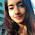thanks for providing ....these imporatant questions

3.this is not important questions these are from exercise !

4.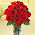thanks for providing these NCERT & Extra solutions............ Thanks again

5.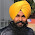thank u for this big help to me in exams

6.it is having extra problems than text and extra information,
it is wonderful........................

1.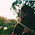2.7.i want other than ncert book

plz

8.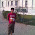i want other than ncert book

plz